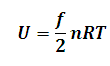# Internal Energy of Ideal Gas Calculator

This Calctown Calculator calculates the internal energy ie. rotational, vibrational and translational kinetic enrgy of ideal gases.

Please note f = 3 for mono-atomic gaese

f = 5 for di-atomic gases

f = 6 for tri-atomic gases

mol
K

#### Result

Jwhere

f = degree of freedom

R =universal gas constant

n = number of moles

T = temperature